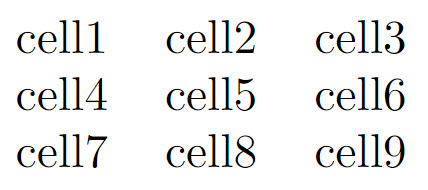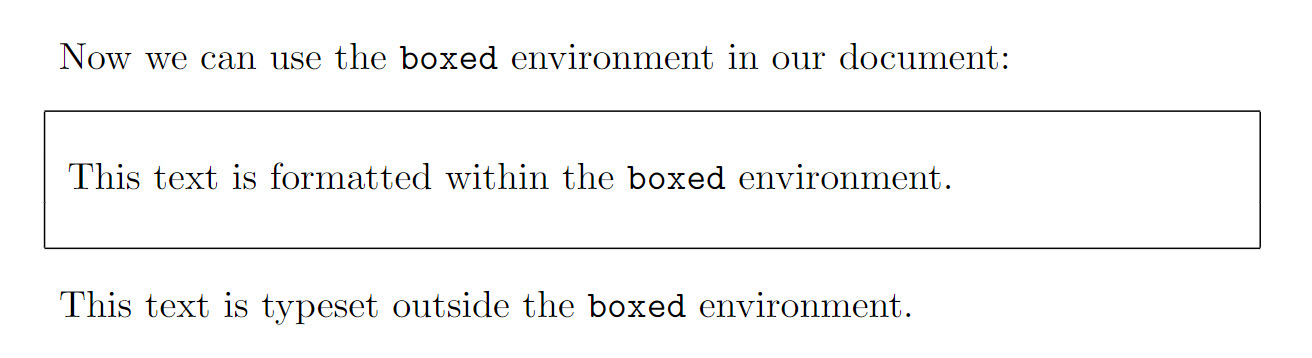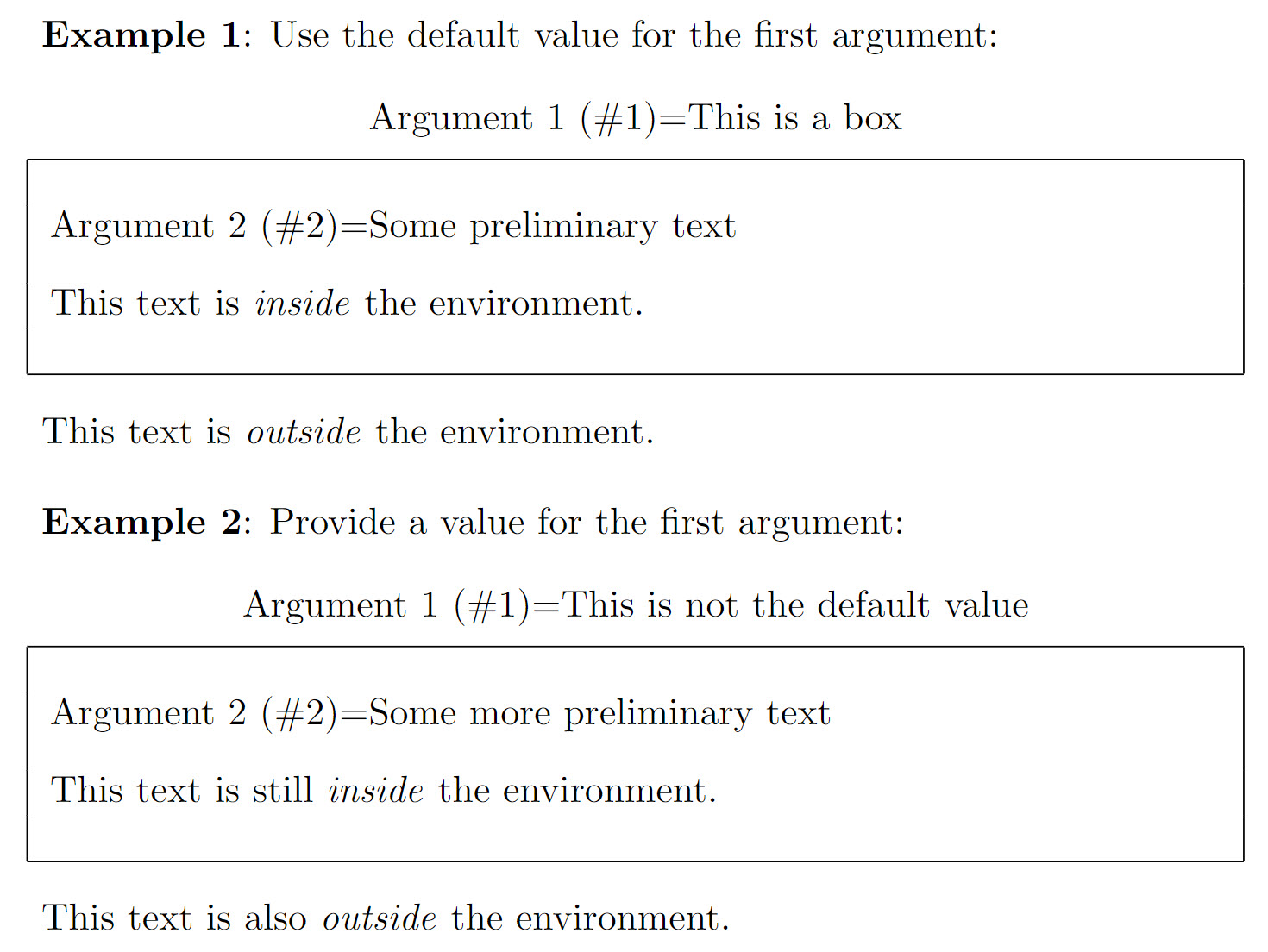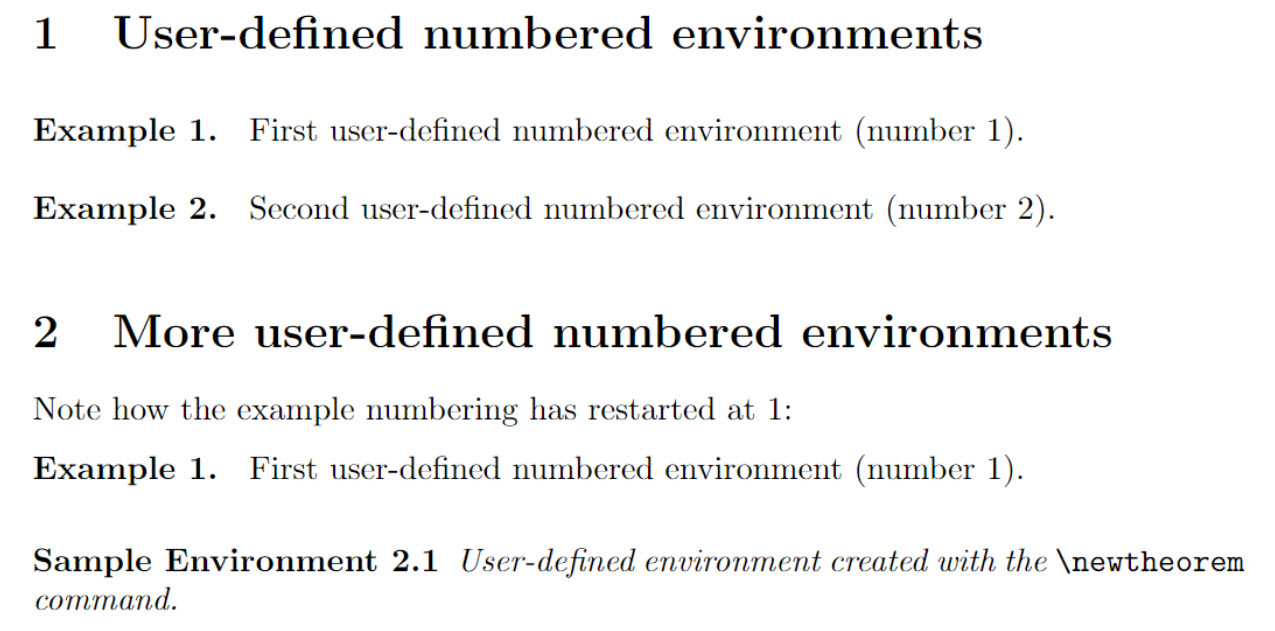LaTeX environments are used to apply specific typesetting effect(s) to a section of your document’s content. This article explains how to use existing environments and define new ones.

## Introduction

An environment starts with \begin{name} and ends with \end{name}:

\begin{name}
...goes here...
\end{name}

where “name” is the name of the environment being used—such as the following example using “center” to format (center) a paragraph of text:

\documentclass{article}
\begin{document}
\begin{center}
This is a demonstration of the \texttt{center} environment.
This paragraph of text will be \textit{centred} because it is
contained within a special environment. Environments provide
an efficient way to modify blocks of text within your document.
\end{center}
\end{document}


This example produces the following output:## Environments

The following example uses the tabular environment to typeset a small table. tabular takes an additional argument { c c c } which defines the alignment of the cells—see the Tables article for more information.

\documentclass{article}
\begin{document}
\begin{tabular}{ c c c }
cell1 & cell2 & cell3 \\
cell4 & cell5 & cell6 \\
cell7 & cell8 & cell9 \\
\end{tabular}
\end{document}


This example produces the following output:Some environments accept optional arguments which are usually passed inside brackets ([...]).

## Defining a new environment

To define a new environment use the \newenvironment command which has the general form:

\newenvironment{name}[numarg][optarg_default]{begin_def}{end_def}

where:

• name is the name of this user-defined argument;
• numarg is the number of arguments, from 1 to 9, this environment accepts. If [numarg] is omitted then the environment does not accept any arguments—such as the boxed environment defined in the next example;
• optarg_default makes the first argument optional and provides a default value—i.e., it is the value used if an optional argument value is not provided;
• begin_def is LaTeX code executed when the environment starts (opens), i.e., when you write \begin{name}. Within this code you can use arguments accepted by the environment—note that the optional argument is #1 and the remaining arguments are accessed using #2 to #numarg;
• end_def is LaTeX code executed when the environment ends (closes); i.e., when you write \end{name}. You cannot use any of the arguments within this code section.

### Defining simple environments

In this first example, we define the boxed environment which does not take any arguments. It draws a box around text contained within the environment:

\documentclass{article}
%We can define the environment in the preamble
\newenvironment{boxed}
{\begin{center}
\begin{tabular}{|p{0.9\textwidth}|}
\hline\\
}
{
\\\\\hline
\end{tabular}
\end{center}
}
%
\begin{document}
Now we can use the \texttt{boxed} environment in our document:

\begin{boxed}
This text is formatted within the \texttt{boxed} environment.
\end{boxed}

This text is typeset outside the \texttt{boxed} environment.
\end{document}


This example produces the following output:If we compare our definition of boxed with the general form of \newenvironment we can see:

• name is boxed;
• neither [numarg] or [optarg_default] are provided because this environment does not take any arguments;
• begin_def is the LaTeX code—provided between a pair of braces {...}—which is executed when the environment starts (opens):

\begin{center}
\begin{tabular}{|p{0.9\textwidth}|}
\hline\\


• end_def is the LaTeX code—provided between a pair of braces {...}—which is executed when the environment stops (closes):

    \\\\\hline
\end{tabular}
\end{center}


In the example, begin_def starts a center environment and within that a tabular environment is opened to draw vertical lines and a horizontal line around the text we place in the environment. end_def draws another horizontal line then closes the tabular and center environments.

### Defining environments which take arguments

Let’s enhance the previous example to do the following:

• use an optional argument to typeset a title. If a title is not provided, use the default value This is a box;
• use a second argument which contains material to typeset ahead of the text we provide within the environment itself.

So we have 2 arguments:

• the first (argument 1) is optional;
• the second (argument 2) is not.

This means numarg=2.

We can use the following definition of our updated boxed environment:

\newenvironment{boxed}[This is a box]
{\begin{center}
Argument 1 (\#1)=#1\\[1ex]
\begin{tabular}{{{!}}p{0.9\textwidth}{{!}}}
\hline\\
Argument 2 (\#2)=#2\\[2ex]
}
{
\\\\\hline
\end{tabular}
\end{center}
}


This enhanced version differs from the original in the following ways:

• [numarg] is present and has the value 2;
• [optarg_default] is also present, making the first argument optional with a default value This is a box.

The following example demonstrates some uses of our upgraded boxed environment:

\documentclass{article}
% Note the default value for the first
% argument is provided by [This is a box]
\newenvironment{boxed}[This is a box]
{\begin{center}
Argument 1 (\#1)=#1\\[1ex]
\begin{tabular}{|p{0.9\textwidth}|}
\hline\\
Argument 2 (\#2)=#2\\[2ex]
}
{
\\\\\hline
\end{tabular}
\end{center}
}
\begin{document}
\textbf{Example 1}: Use the default value for the first argument:

\begin{boxed}{Some preliminary text}
This text is \textit{inside} the environment.
\end{boxed}

This text is \textit{outside} the environment.

\vskip12pt

\textbf{Example 2}: Provide a value for the first argument:

\begin{boxed}[This is not the default value]{Some more preliminary text}
This text is still \textit{inside} the environment.
\end{boxed}

This text is also \textit{outside} the environment.
\end{document}


This example produces the following output:### Numbered environments

Numbered environments can be created either manually or directly with the command \newtheorem, as demonstrated by the following example:

\documentclass{article}
% Define our numbered environment within the preamble
\newcounter{example}[section]
\newenvironment{example}[]{\refstepcounter{example}\par\medskip
\noindent \textbf{Example~\theexample. #1} \rmfamily}{\medskip}

% Another numbered environment defined with \newtheorem
\usepackage{amsmath} % For the \newtheorem command
\newtheorem{SampleEnv}{Sample Environment}[section]
\begin{document}

\section{User-defined numbered environments}

\begin{example}
First user-defined numbered environment (number \theexample).
\end{example}

\begin{example}
Second user-defined numbered environment (number \theexample).
\end{example}

\section{More user-defined numbered environments}
Note how the example numbering has restarted at 1:

\begin{example}
First user-defined numbered environment (number \theexample).
\end{example}

\begin{SampleEnv}
User-defined environment created with the \verb|\newtheorem| command.
\end{SampleEnv}
\end{document}


This example produces the following output:#### Some notes

• In the manually-defined example environment the command \newcounter{example}[section] creates a counter, also called example. The example counter is:
• incremented by 1 by using \refstepcounter{example} within the environment definition;
• reset every time a new \section{...} is started;
• a variable whose current value can be typeset using \theexample. See the article about counters to learn more.
• The command \newtheorem directly creates a numbered environment and takes three parameters:
• the name of the new environment: SampleEnv in our example;
• the text to be printed in bold at the beginning of the line: Sample Environment in our example;
• an optional parameter which determines when the counter is reset; and if it’s used the counter gets preceded by that reset counter’s value: section in our example.

The amsthm package, along with amsmath, provide useful extra definitions alongside \newtheorem; see Theorems and proofs for further details.

## Redefining existing environments

Environments can be redefined using \renewenvironment whose syntax is the same as \newenvironment—see the section Defining a new environment for an explanation of the various parameters:

\newenvironment{name}[numarg][optarg_default]{begin_def}{end_def}

The next example redefines the itemize environment—this is only an example to illustrate the process, it should not be considered within a real document. The new itemize environment no longer produces a bulleted list; instead, it centres and emphasizes (italicizes) the text within it (using the \em command).

\documentclass{article}
% Redefine the environment in the preamble
\renewenvironment{itemize}
{\begin{center}\em}
{\end{center}}
\begin{document}

\begin{itemize}
We have redefined the \texttt{itemize} environment so that any text
within it is centred and emphasised (italicized). It no longer creates
a bulleted list---this is only an example and not intended for use
in real documents!
\end{itemize}
\end{document}


This example produces the following output: